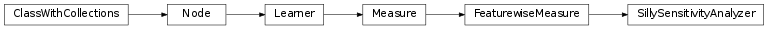mvpa2.testing.clfs¶

Provides clfs dictionary with instances of all available classifiers.Classes

 Classifier([space]) Abstract classifier class to be inherited by all classifiers Dataset(samples[, sa, fa, a]) Generic storage class for datasets with multiple attributes. FeaturewiseMeasure([null_dist]) A per-feature-measure computed from a Dataset (base class). Less1Classifier(\*\*kwargs) Dummy classifier which reports +1 class if abs value of max less than 1 LinearCSVMC([C]) C-SVM classifier using linear kernel. LinearNuSVMC([nu]) Nu-SVM classifier using linear kernel. LinearSVMKernel alias of LinearLSKernel RandomClassifier(\*\*kwargs) Dummy classifier deciding on labels absolutely randomly RbfCSVMC([C]) C-SVM classifier using a radial basis function kernel RbfNuSVMC([nu]) Nu-SVM classifier using a radial basis function kernel RbfSVMKernel alias of RbfLSKernel SMLR(\*\*kwargs) Sparse Multinomial Logistic Regression Classifier. SVM(\*\*kwargs) Support Vector Machine Classifier. SameSignClassifier(\*\*kwargs) Dummy classifier which reports +1 class if both features have SillySensitivityAnalyzer([mult]) Simple one which just returns xrange[-N/2, N/2], where N is the kNN([k, dfx, voting]) k-Nearest-Neighbour classifier.